# Test: Z-Transform

## 10 Questions MCQ Test Topicwise Question Bank for Electrical Engineering | Test: Z-Transform

Description
Attempt Test: Z-Transform | 10 questions in 30 minutes | Mock test for Electrical Engineering (EE) preparation | Free important questions MCQ to study Topicwise Question Bank for Electrical Engineering for Electrical Engineering (EE) Exam | Download free PDF with solutions
QUESTION: 1

Solution:
QUESTION: 2

Solution:
QUESTION: 3

### The z-transform of a signal is given by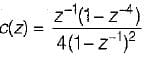Its final valve is

Solution:
QUESTION: 4

Which one of the following represents the impulse response of a system defined by H(z) = z-m

Solution:
QUESTION: 5

The z-transform of the time function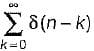is

Solution:
QUESTION: 6

The z-transform of the system is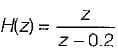if the ROC is |z| < 0.2, then the impulse response of the system is

Solution:
QUESTION: 7

If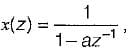the two discrete time signal

Solution:
QUESTION: 8

lf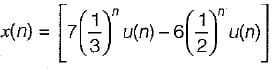The ROC for the above discrete time signal is

Solution:
QUESTION: 9

A stable and causal system is described by the difference equation,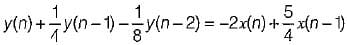The impulses response of the system is

Solution:
QUESTION: 10

A linear discrete time system has the characteristics equation, z3 - 0.81 z = 0, the system is

Solution:Use Code STAYHOME200 and get INR 200 additional OFF Use Coupon Code